# Chapter 5 The Second Law of Thermodynamics continued

• Slides: 23Chapter 5 The Second Law of Thermodynamics (continued)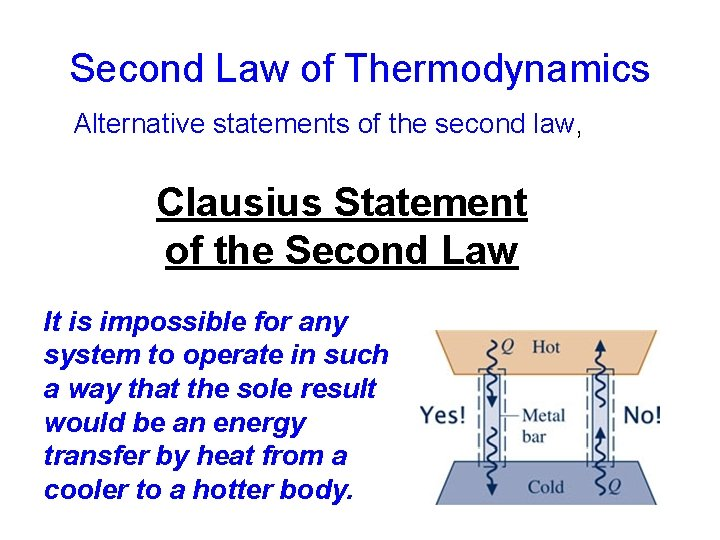Second Law of Thermodynamics Alternative statements of the second law, Clausius Statement of the Second Law It is impossible for any system to operate in such a way that the sole result would be an energy transfer by heat from a cooler to a hotter body.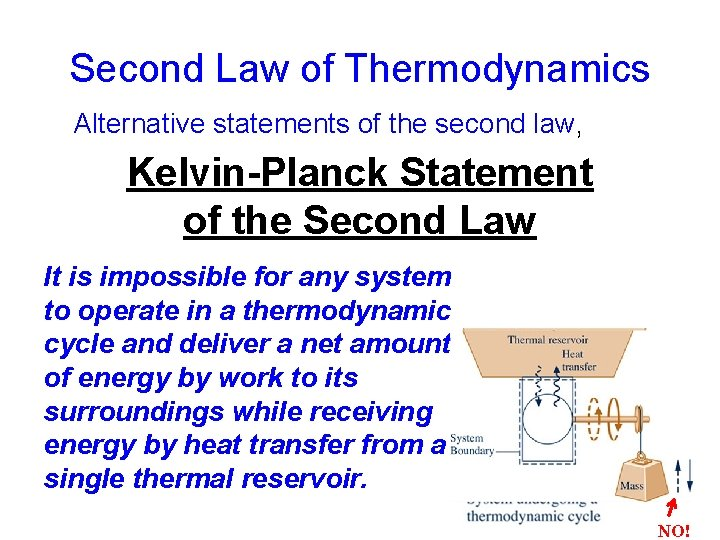Second Law of Thermodynamics Alternative statements of the second law, Kelvin-Planck Statement of the Second Law It is impossible for any system to operate in a thermodynamic cycle and deliver a net amount of energy by work to its surroundings while receiving energy by heat transfer from a single thermal reservoir. NO!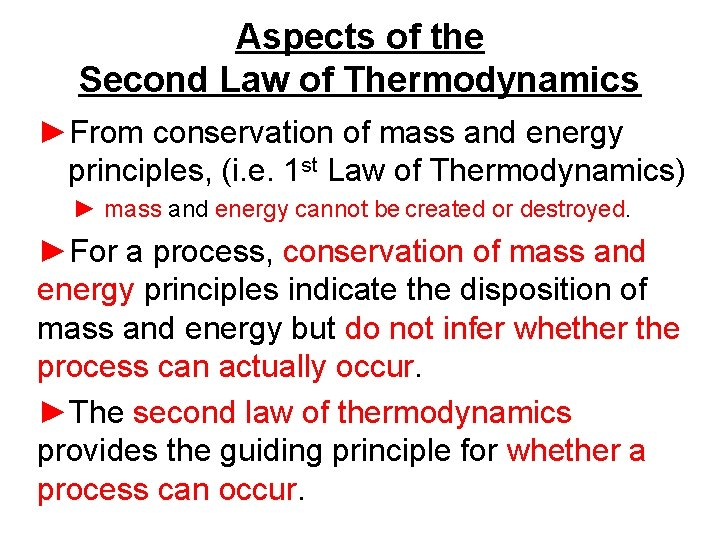Aspects of the Second Law of Thermodynamics ►From conservation of mass and energy principles, (i. e. 1 st Law of Thermodynamics) ► mass and energy cannot be created or destroyed. ►For a process, conservation of mass and energy principles indicate the disposition of mass and energy but do not infer whether the process can actually occur. ►The second law of thermodynamics provides the guiding principle for whether a process can occur.Second Law of Thermodynamics Alternative Statements There is no simple statement that captures all aspects of the second law. Several alternative formulations of the second law are found in the technical literature. Three prominent ones are: ►Clausius Statement ►Kelvin-Planck Statement ►Entropy Statement It is impossible for any system to operate in a way that entropy is destroyed.Irreversible and Reversible Processes During a process (i. e. a change from State A to State B) of a system, irreversibilities may be present: ►within the system, or ►with its surroundings (usually the immediate surroundings), or ►both.Applications to Power Cycles Interacting with Two Thermal Reservoirs For a system undergoing a power cycle while communicating thermally with two thermal reservoirs, a hot reservoir and a cold reservoir, thermal efficiency of any such cycle is (Eq. 5. 4)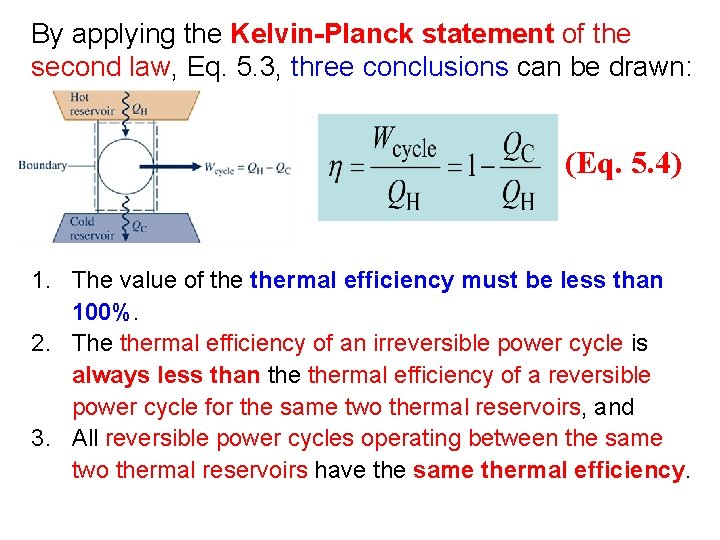By applying the Kelvin-Planck statement of the second law, Eq. 5. 3, three conclusions can be drawn: (Eq. 5. 4) 1. The value of thermal efficiency must be less than 100%. 2. The thermal efficiency of an irreversible power cycle is always less than thermal efficiency of a reversible power cycle for the same two thermal reservoirs, and 3. All reversible power cycles operating between the same two thermal reservoirs have the same thermal efficiency.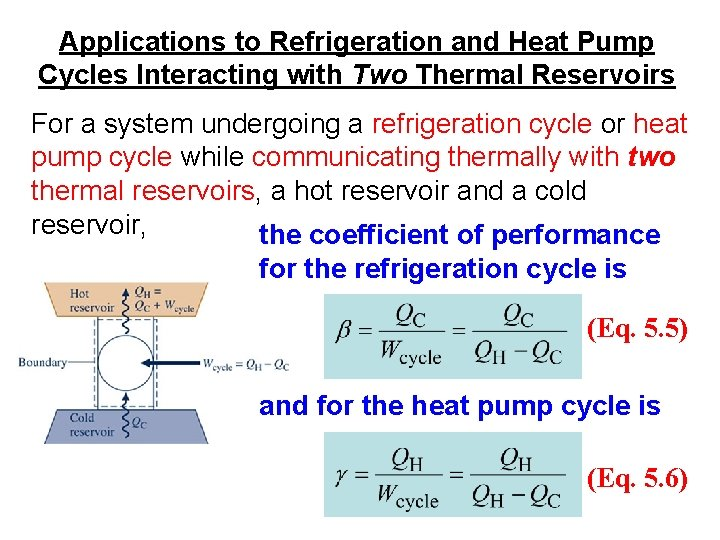Applications to Refrigeration and Heat Pump Cycles Interacting with Two Thermal Reservoirs For a system undergoing a refrigeration cycle or heat pump cycle while communicating thermally with two thermal reservoirs, a hot reservoir and a cold reservoir, the coefficient of performance for the refrigeration cycle is (Eq. 5. 5) and for the heat pump cycle is (Eq. 5. 6)By applying the Kelvin-Planck statement of the second law, Eq. 5. 3, three conclusions can be drawn: 1. For a refrigeration effect to occur a net work input Wcycle is required. Accordingly, the coefficient of performance must be finite in value. 2. The coefficient of performance of an irreversible refrigeration cycle is always less than the coefficient of performance of a reversible refrigeration cycle when each operates between the same two thermal reservoirs. 3. All reversible refrigeration cycles operating between the same two thermal reservoirs have the same coefficient of performance.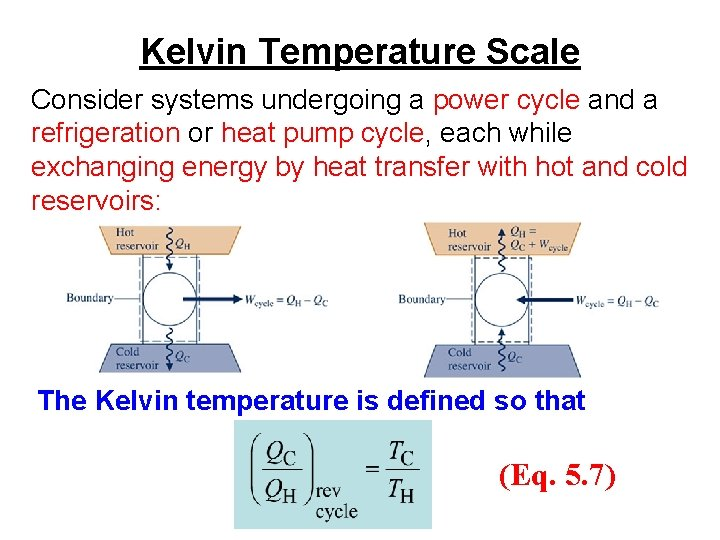Kelvin Temperature Scale Consider systems undergoing a power cycle and a refrigeration or heat pump cycle, each while exchanging energy by heat transfer with hot and cold reservoirs: The Kelvin temperature is defined so that (Eq. 5. 7)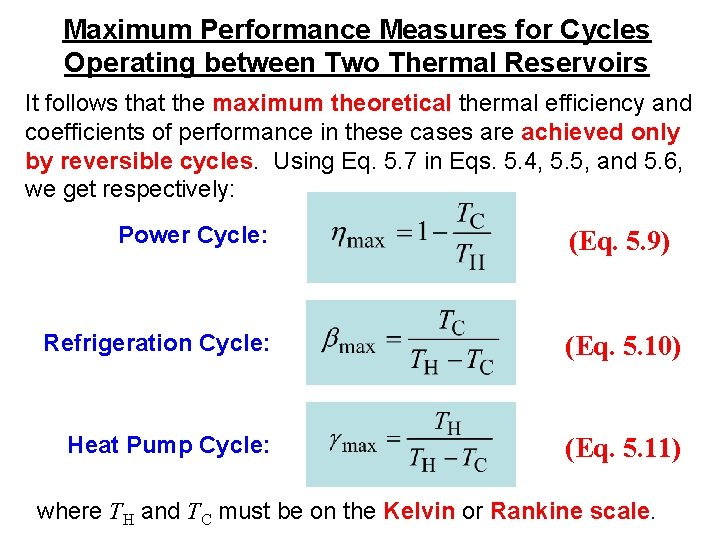Maximum Performance Measures for Cycles Operating between Two Thermal Reservoirs It follows that the maximum theoretical thermal efficiency and coefficients of performance in these cases are achieved only by reversible cycles. Using Eq. 5. 7 in Eqs. 5. 4, 5. 5, and 5. 6, we get respectively: Power Cycle: (Eq. 5. 9) Refrigeration Cycle: (Eq. 5. 10) Heat Pump Cycle: (Eq. 5. 11) where TH and TC must be on the Kelvin or Rankine scale.Example: Power Cycle Analysis A system undergoes a power cycle while receiving 1000 k. J by heat transfer from a thermal reservoir at a temperature of 500 K and discharging 600 k. J by heat transfer to a thermal reservoir at (a) 200 K, (b) 300 K, (c) 400 K. For each case, determine whether the cycle operates irreversibly, operates reversibly, or is impossible. Solution: To determine the nature of the cycle, compare actual cycle performance (h) to maximum theoretical cycle performance (hmax) calculated from Eq. 5. 9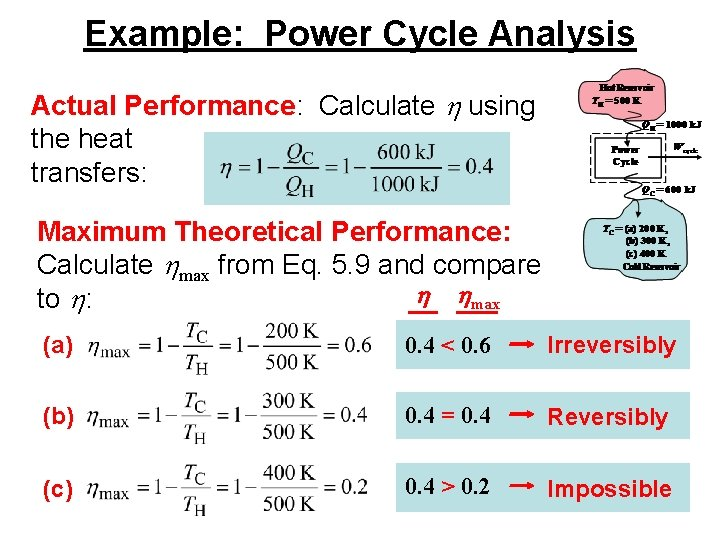Example: Power Cycle Analysis Actual Performance: Calculate h using the heat transfers: Maximum Theoretical Performance: Calculate hmax from Eq. 5. 9 and compare h hmax to h: (a) 0. 4 < 0. 6 Irreversibly (b) 0. 4 = 0. 4 Reversibly (c) 0. 4 > 0. 2 ImpossibleCarnot Cycle ►The Carnot cycle provides a specific example of a reversible cycle that operates between two thermal reservoirs. Other examples are provided in Chapter 9: the Ericsson and Stirling cycles. ►In a Carnot cycle, the system executing the cycle undergoes a series of four internally reversible processes: two adiabatic processes alternated with two isothermal processes.Carnot Power Cycles The p-v diagram and schematic of a gas in a piston-cylinder assembly executing a Carnot cycle are shown below:Carnot Power Cycles The p-v diagram and schematic of water executing a Carnot cycle through four interconnected components are shown below: In each of these cases thermal efficiency is given by (Eq. 5. 9)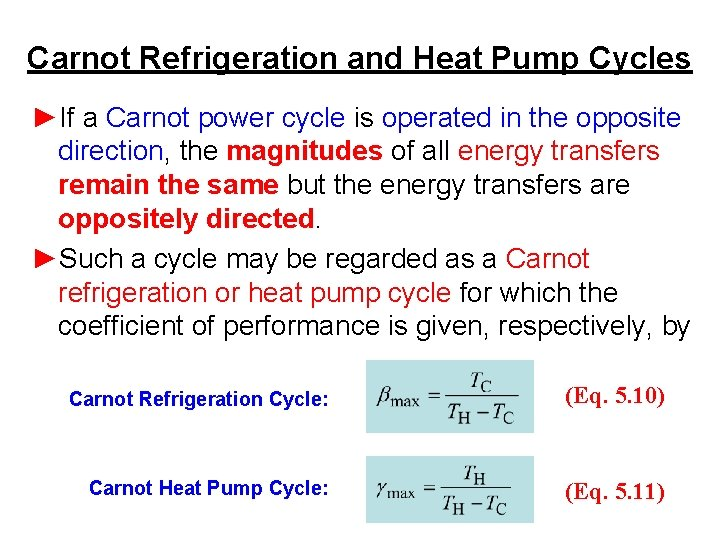Carnot Refrigeration and Heat Pump Cycles ►If a Carnot power cycle is operated in the opposite direction, the magnitudes of all energy transfers remain the same but the energy transfers are oppositely directed. ►Such a cycle may be regarded as a Carnot refrigeration or heat pump cycle for which the coefficient of performance is given, respectively, by Carnot Refrigeration Cycle: (Eq. 5. 10) Carnot Heat Pump Cycle: (Eq. 5. 11)Clausius Inequality ►The Clausius inequality considered next provides the basis for developing the entropy concept in Chapter 6. ►The Clausius inequality is applicable to any cycle without regard for the body, or bodies, from which the system undergoing a cycle receives energy by heat transfer or to which the system rejects energy by heat transfer. Such bodies need not be thermal reservoirs.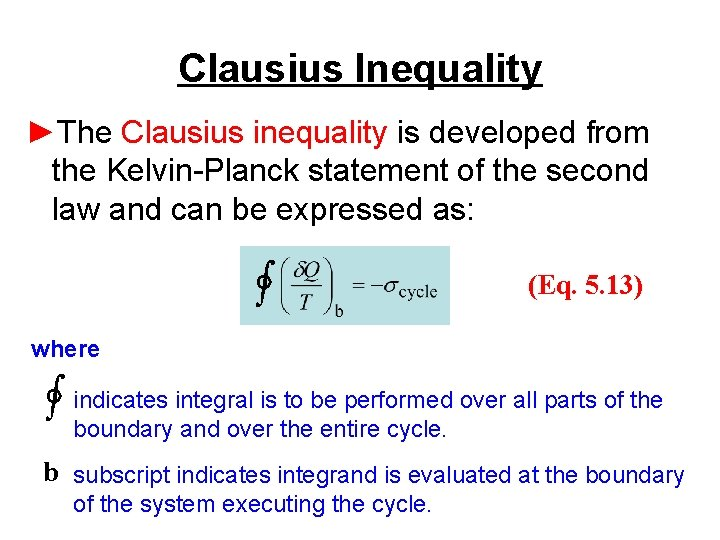Clausius Inequality ►The Clausius inequality is developed from the Kelvin-Planck statement of the second law and can be expressed as: ∫ (Eq. 5. 13) where ∫ indicates integral is to be performed over all parts of the boundary and over the entire cycle. b subscript indicates integrand is evaluated at the boundary of the system executing the cycle.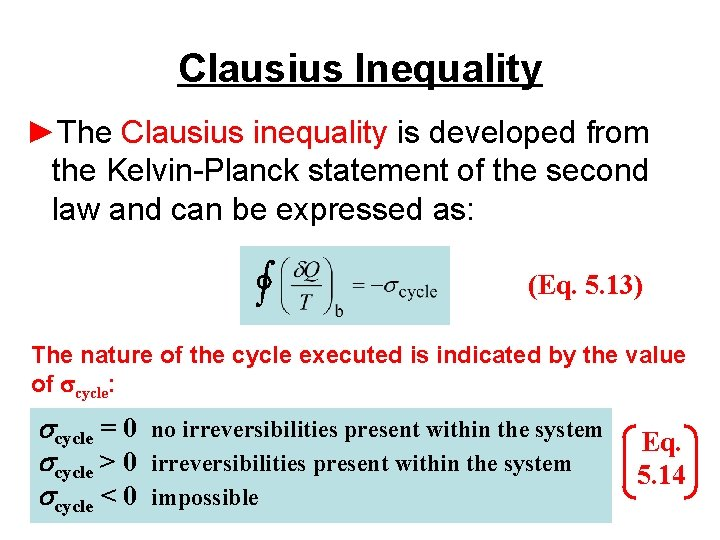Clausius Inequality ►The Clausius inequality is developed from the Kelvin-Planck statement of the second law and can be expressed as: ∫ (Eq. 5. 13) The nature of the cycle executed is indicated by the value of scycle: scycle = 0 no irreversibilities present within the system scycle > 0 irreversibilities present within the system scycle < 0 impossible Eq. 5. 14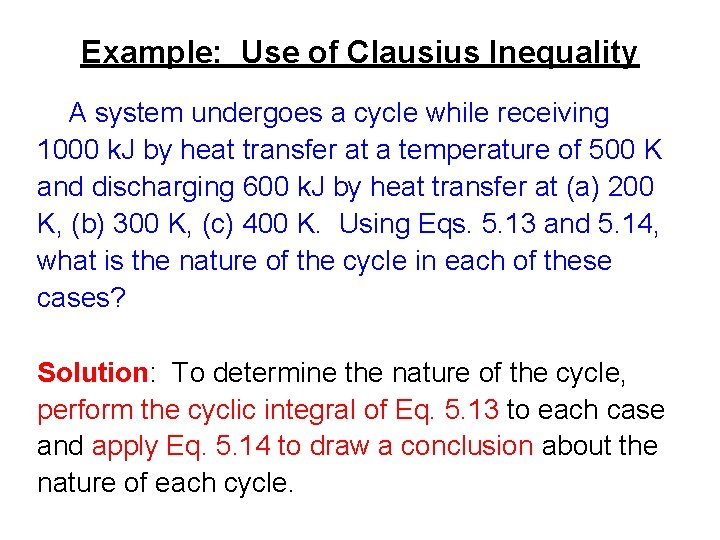Example: Use of Clausius Inequality A system undergoes a cycle while receiving 1000 k. J by heat transfer at a temperature of 500 K and discharging 600 k. J by heat transfer at (a) 200 K, (b) 300 K, (c) 400 K. Using Eqs. 5. 13 and 5. 14, what is the nature of the cycle in each of these cases? Solution: To determine the nature of the cycle, perform the cyclic integral of Eq. 5. 13 to each case and apply Eq. 5. 14 to draw a conclusion about the nature of each cycle.Example: Use of Clausius Inequality Applying Eq. 5. 13 to each cycle: (a) ∫ scycle = +1 k. J/K > 0 Irreversibilities present within system (b) scycle = 0 k. J/K = 0 No irreversibilities present within system (c) scycle = – 0. 5 k. J/K < 0 Impossible